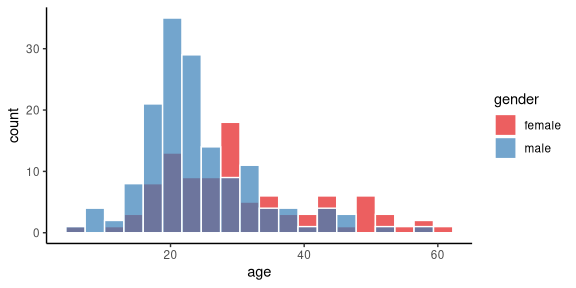# Introduction

R provides very powerful tools for data visualization, particularly ggplot. This leads to a dilemma when teaching, however. Do we first teach the basics or ggplot before students can visualize their data, or do we use other simpler tools for summarization and visualization, albeit of a limited kind, and later teach more about the powerful ggplot tools? The psyntur package aims to provide some tools if the latter approach is taken. These functions aim to make it quick and easy to perform the common kinds of data exploration and visualization. All functions, however, are wrappers around sets of ggplot commands In this vignette, we showcase some of these tools.

The required functions as well as some example psychological science data sets can be loaded with the usual library command.

library(psyntur)

# Scatterplots

We can perform a standard 2d scatterplot with scatterplot. For example, using the faithfulfaces data provided by psyntur, we can plot a scatterplot showing the relationship between the perceived (sexual) faithfulness of a person in a photo against their perceived trustworthiness as follows.

scatterplot(x = trustworthy, y = faithful, data = faithfulfaces)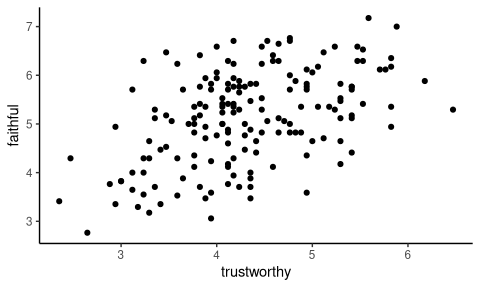We can colour code the points according to the value of a third, usually categorical variable, by using the by argument. For example, here we colour code the points according to the sex of the person in the photo.

scatterplot(x = trustworthy, y = faithful, data = faithfulfaces, by = face_sex)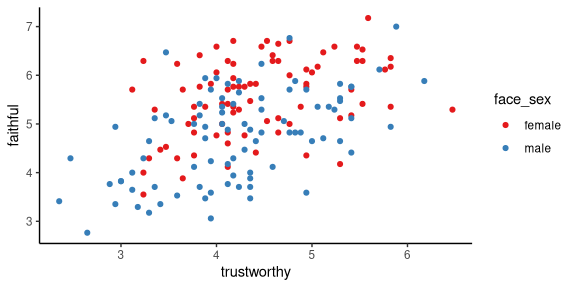We can add the line of best fit to all points in the scatterplot, or to each set of coloured points in the scatterplot if by is used, with the best_fit_line argument.

scatterplot(x = trustworthy, y = faithful, data = faithfulfaces,
by = face_sex, best_fit_line = TRUE)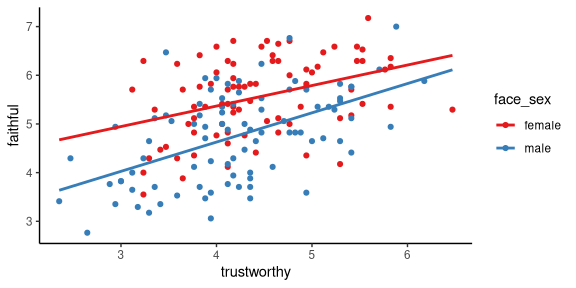# Boxplots

Boxplots, also known as box-and-whisker plots, or Tukey boxplots or box-and-whisker plots, display the distributions of univariate data, optionally grouped according to other variables. For example, to show a boxplot of the distribution of all the response times in an experiment that collected response times in different conditions, which is provided by the vizverb data sets, we can do the following.

tukeyboxplot(y = time, data = vizverb)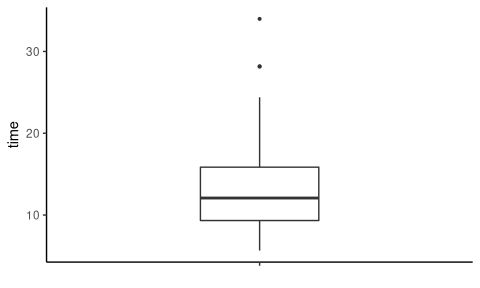We can add all the points as jittered points as follows.

tukeyboxplot(y = time, data = vizverb, jitter = TRUE)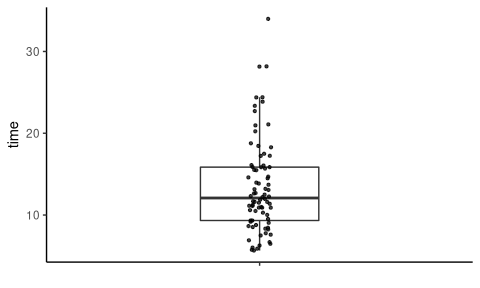If we want to plot the distribution of time for each of the two different tasks performed in the experiment, provide by the task variable in vizverb, we can set the x variable to task as follows.

tukeyboxplot(y = time, x= task, data = vizverb)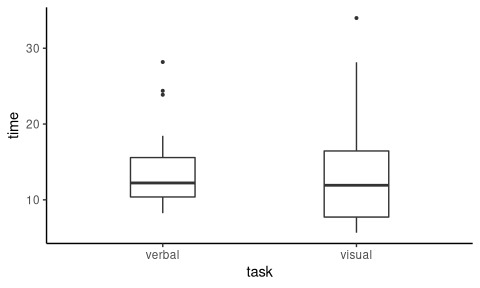The vizverb data is from a two-way factorical experiment where reaction times are collected for different tasks (the task variable) and using different responses (the response variable). We can plot the distribution of time according to both task and response by using x and by together as follows.

tukeyboxplot(y = time, x= task, data = vizverb, by = response)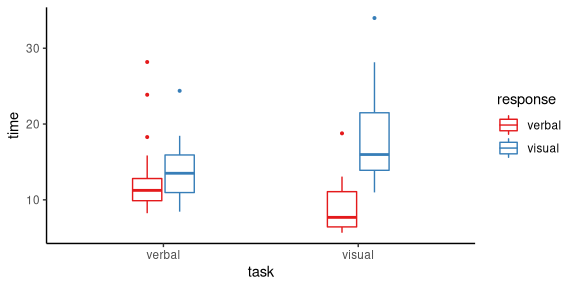Jittered points can be put on these plots by using jitter = TRUE.

tukeyboxplot(y = time, x= task, data = vizverb,
by = response, jitter = TRUE)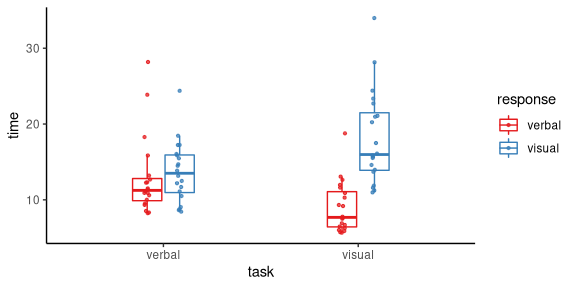Continuous variables can be used as the x variable as in the following example using the R built in ToothGrowth data set.

tukeyboxplot(y = len, x = dose, data = ToothGrowth)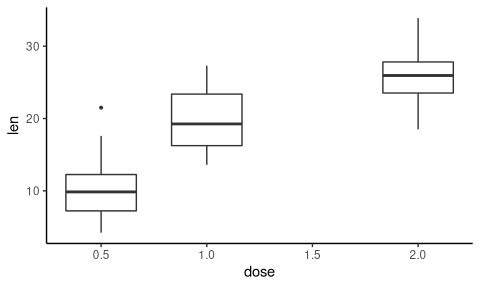This can be used with by and jitter too.

tukeyboxplot(y = len, x = dose, data = ToothGrowth,
by = supp, jitter = TRUE, jitter_width = .5)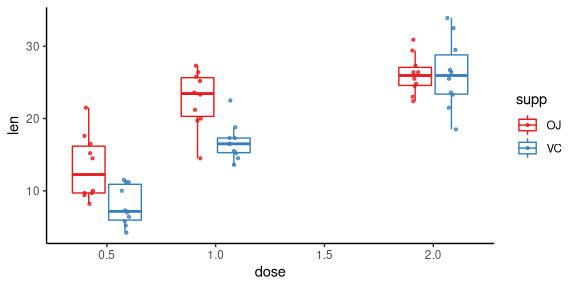# Histograms

Histograms can be made with the histrogram function. For example, to plot the onset ages of schizophrenia, using the schizophrenia data, we can do the following.

histogram(x = age, data = schizophrenia)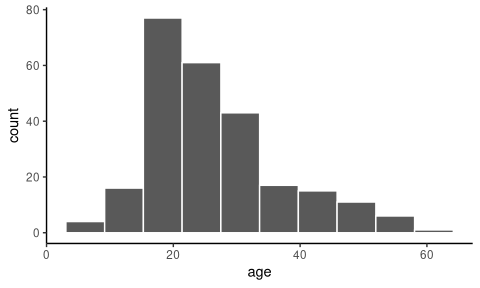We can change the number of bins from its default of 10 with the bins argument.

histogram(x = age, data = schizophrenia, bins = 20)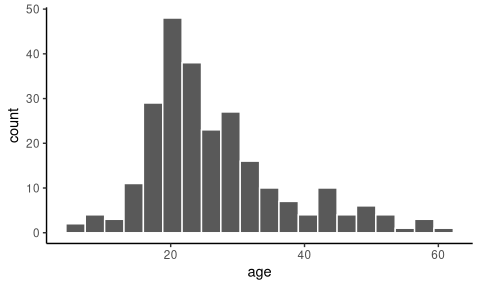If we use a grouping variable, such as the gender variable, using the by argument, we obtain a stacked histogram.

histogram(x = age, data = schizophrenia, by = gender, bins = 20)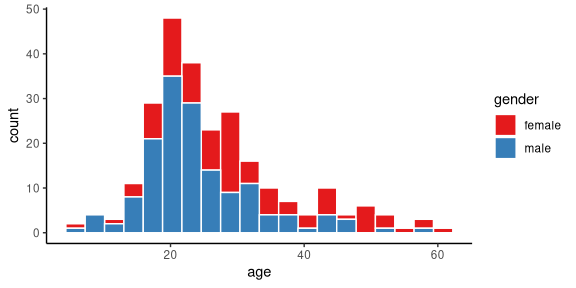When using by, we can obtain dodged rather than stacked histograms by setting position = 'dodge' as in the following example.

histogram(x = age, data = schizophrenia,
by = gender, bins = 20, position = 'dodge')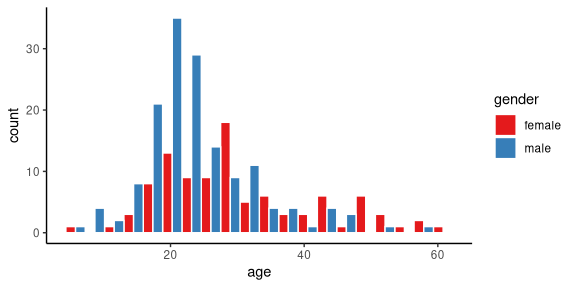Likewise, we can obtain overlapping histograms by setting position = 'identity' as in the following example.

histogram(x = age, data = schizophrenia,
by = gender, bins = 20, position = 'identity')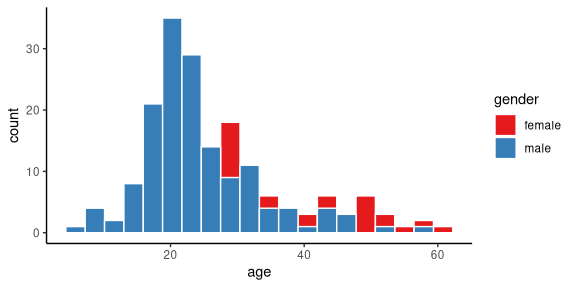In the case of position = 'identity', it is usually required to make the bars transparent by setting the alpha value to be less than 1.0, as in the following example.

histogram(x = age, data = schizophrenia,
by = gender, bins = 20, position = 'identity', alpha = 0.7)